×#### Thank you for registering.

One of our academic counsellors will contact you within 1 working day.

Click to Chat

1800-1023-196

+91-120-4616500

CART 0

• 0

MY CART (5)

Use Coupon: CART20 and get 20% off on all online Study Material

ITEM
DETAILS
MRP
DISCOUNT
FINAL PRICE
Total Price: Rs.

There are no items in this cart.
Continue Shopping```21:       Area bounded by y = g(x), x-axis and the lines x = -2, x = 3, whereand f(x) = x2 -  , is equal to

(A)  113/24 sq.units                       (B)         111/24 sq.units

(C)  117/24 sq.units                       (D)         121/24 sq,units22:                  Area of the region which consists of all the points satisfying the conditions |x–y| + |x+y| < 8 and xy > 2, is equal to

(A)      4(7 – ln8)sq. units               (B)      4(9 – ln8)sq. units

(C)      2(7 – ln8)sq. units               (D)      2(9 – ln8)sq. units

Solution:       The expression |x–y| + |x+y| < 8, represents the interior region of the square formed by the lines x = ± 4, y = ± 4 and xy > 2. represents the region lying inside the hyperbola xy =2Required area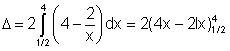= 4(7–3 In2) sq.units

= 4(7 – In8) sq.units

23:                  Area bounded by the parabola (y - 2)2 = x – 1, the tangent to it at the point P (2, 3) and the x-axis is equal to

(A)      9 sq. units                             (B)      6 sq. units

(C)      3 sq. units                             (D)      None of these

Solution:       (y - 2)2 = (x - 1) => 2(y - 2).  = 1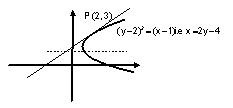=> dy/dx = 1/2(y–2)

Thus equation of tangent at P(2, 3) is,

(y – 3) = 1/2 (x–2) i.e., x = 2y – 4

Required area= ((y–2)3/3 – y2 + 5y)30 = 9 sq. units

24:                  Two lines draw through the point P(4, 0) divide the area bounded by the curves y = √2 πx/4 and x – axis, between the linea x = 2, and x = 4, in to three equal parts. Sum of the slopes of the drawn lines is equal to

(A) –2 2/π                                     (B)      –√2/π

(C) –√2/π                                     (D)      –4√2/π

Solution:       Area bounded by y = √2 .sin πx/4 and x-axis between the lines x = 2 and x = 4,Let the drawn lines are L1: y – m1(x - 4) = 0 and L2: y – m2(x - 4) = 0, meeting the line x = 2 at the points A and B respectively Clearly A = (2, - 2m1); B= (2, -2m2)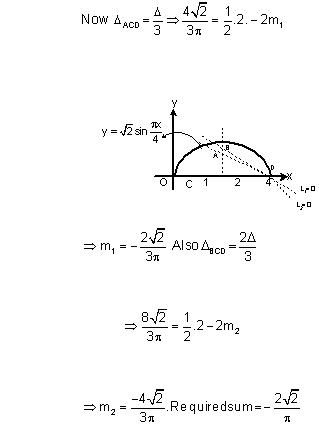25:                  If A =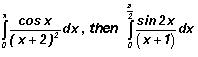is equal to

(A)   1/π+2 – A                               (B)      1/2 + 1/π+2 – A

(C)   1/2 – 1/π+2 – A                     (D)      1/2 + 1/π+2 + A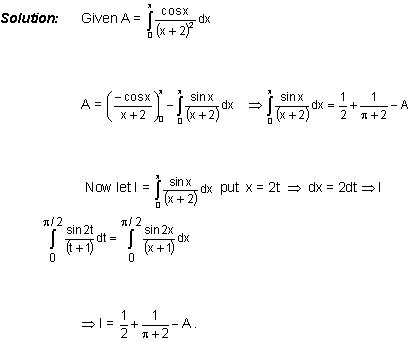26.                   The value of the integral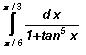is

(A) 1                                                                         (B) π/12

(C) π/6                                                                     (D) none of these

Solution:       Using the propertyf (a + b – x) dx, the given integral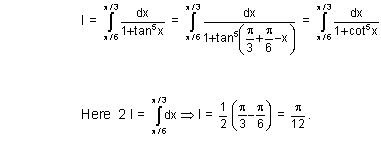Hence (B) is the correct answer.

27.                   If I =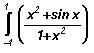dx then

(A) 0                                                               (B) 2

(C)  π/2                                                         (D) 2 – π/2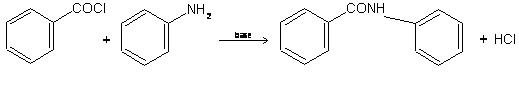Hence (D) is the correct answer.

28.                   If I =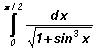, then

(A) 0 < I < 1                                                   (B) I > π/2

(C) I < √2π                                                     (D) I > 2 π

Solution:       Since x ∈ [0, π/2]  => 1 < 1 + sin3 x < 2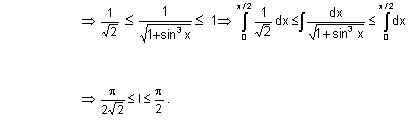Hence (C) is the correct answer.

29.                   If f (a + b –x) = f (x) then ∫ba x f (x) dx is equal to

(A) a–b/2 ∫ba f(x) dx                                    (B) (a+b/2) ∫ba f(x) d x

(C) 0                                                              (D) none of these

Solution:        I = ∫ba x f (x) dx = ∫ba (a+b-x) f (a +b-x) dx

= (a + b) ∫ba  f(a +b –x) - ∫ba x f (a + b –x) dx

= ( a + b) ∫ba f (x) dx - ∫ba x f (x) d x

Hence I = (a+b/2) ∫ba f(x) dx.

Hence (B) is the correct answer.

To read more, Buy study materials of Definite integral comprising study notes, revision notes, video lectures, previous year solved questions etc. Also browse for more study materials on Mathematics here.
```### Course Features

• 731 Video Lectures
• Revision Notes
• Previous Year Papers
• Mind Map
• Study Planner
• NCERT Solutions
• Discussion Forum
• Test paper with Video Solution